# 1 Introduction

The Standard Model of particle physics is one of the greatest triumphs of physics. This theory is our best attempt to describe all the particles and all the forces of nature... except gravity. It does a great job of fitting experiments we can do in the lab. But physicists are dissatisfied with it. There are three main reasons. First, it leaves out gravity: that force is described by Einstein's theory of general relativity, which has not yet been reconciled with the Standard Model. Second, astronomical observations suggest that there may be forms of matter not covered by the Standard Model--most notably, dark matter'. And third, the Standard Model is complicated and seemingly arbitrary. This goes against the cherished notion that the laws of nature, when deeply understood, are simple and beautiful.

For the modern theoretical physicist, looking beyond the Standard Model has been an endeavor both exciting and frustrating. Most modern attempts are based on string theory. There are also other interesting approaches, such as loop quantum gravity and theories based on noncommutative geometry. But back in the mid 1970's, before any of the currently popular approaches rose to prominence, physicists pursued a program called grand unification'. This sought to unify the forces and particles of the Standard Model using the mathematics of Lie groups, Lie algebras, and their representations. Ideas from this era remain influential, because grand unification is still one of the most fascinating attempts to find order and beauty lurking within the Standard Model.

This paper is a gentle introduction to the group representations that describe particles in the Standard Model and the most famous grand unified theories. To make the material more approachable for mathematicians, we limit our scope by not discussing particle interactions or symmetry breaking'--the way a theory with a large symmetry group can mimic one with a smaller group at low energies. These topics lie at the very heart of particle physics. But by omitting them, we can focus on ideas from algebra that many mathematicians will find familiar, while introducing the unfamiliar ways that physicists use these ideas.

In fact, the essential simplicity of the representation theory involved in the Standard Model and grand unified theories is quite striking. The usual textbook approach to particle physics proceeds through quantum field theory and gauge theory. While these subjects are very important to modern mathematics, learning them is a major undertaking. We have chosen to focus on the algebra of grand unified theories because many mathematicians have the prerequisites to understand it with only a little work.

A full-fledged treatment of particle physics requires quantum field theory, which uses representations of a noncompact Lie group called the Poincaré group on infinite-dimensional Hilbert spaces. This brings in a lot of analytical subtleties, which make it hard to formulate theories of particle physics in a mathematically rigorous way. In fact, no one has yet succeeded in doing this for the Standard Model. But by neglecting the all-important topic of particle interactions, we can restrict attention to finite-dimensional Hilbert spaces: that is, finite-dimensional complex inner product spaces. This makes our discussion purely algebraic in flavor.

Every theory we consider has an internal symmetry group' or gauge group'. This is a compact Lie group, say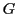. Particles then live in representations ofon a finite-dimensional Hilbert space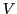. More precisely:can always be decomposed as a direct sum of irreducible representations, or irreps--and for our limited purposes, particles are basis vectors of irreps. This provides a way to organize particles, which physicists have been exploiting since the 1960s.

The idea of unification' has a clear meaning in these terms. Supposeis a representation, not only of, but also some larger group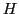havingas a subgroup. Then we expectto decompose into fewer irreps as a representation ofthan as a representation of, because elements ofcan mix different irreps of. So: by introducing a larger symmetry group, particles can be unified into larger irreps.

Grand unification' occurs when the compact Lie groupis simple, and thus not a product of other groups. A gauge theory based onrequires an invariant inner product on its Lie algebra. Whenis simple, this form is unique up to a scale factor, which physicists call a coupling constant': this measures the strength of the force corresponding to. Whenis the product of simple factors, there is one coupling constant for each factor of. So, by using a simple Lie group as gauge group, we minimize the number of coupling constants.

In this paper, we give an account of the algebra behind the Standard Model and three attempts at unification: Georgi and Glashow's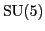theory, Georgi's theory based on the group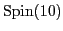(physicists call this the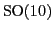theory), and the Pati-Salam model. All three date to around 1974. The first two are known as grand unified theories, or GUTs, because they are based on simple Lie groups. The Pati-Salam model is different: while it is called a GUT by some authors, and does indeed involve unification, it is based on the Lie group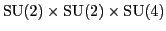, which is merely semisimple.

It is important to note that these theories have their problems. Thetheory predicts that protons will decay more quickly than they do, and it requires certain trends to hold among the relative strengths of forces at high energies--trends which the data do not support. Thetheory may still be viable, especially if at low enough energies it reduces to the Pati-Salam model. However, the issues involved are complex. For details, see the paper by Bertolini et al and the many references therein .

Nonetheless, it is still very much worthwhile for mathematicians to study the algebra of grand unified theories. First, even apart from their physical significance, these theories are intrinsically beautiful mathematical structures. Second, they provide a nice way for mathematicians to get some sense of the jigsaw puzzle that physicists are struggling to solve. It is certainly hopeless trying to understand what physicists are trying to accomplish with string theory without taking a look at grand unified theories. Finally, grand unified theories can be generalized by adding supersymmetry'--and the resulting generalizations are considered serious contenders for describing the real world. For some recent overviews of their prospects, see Pati [26,27] and Peskin .

This is how we shall proceed. In Section 2 we start by describing the Standard Model. After a brief nod to the electron and photon, we explain some nuclear physics in Section 2.1. We start with Heisenberg's old attempt to think of the proton and neutron as two states of a single particle, the nucleon', described by a 2-dimensional representation of. The idea of unification through representation theory traces its origins back to this notion.

After this warmup we tour the Standard Model in its current form. In Section 2.2 we describe the particles called fundamental fermions', which constitute matter. In Section 2.3 we describe the particles called gauge bosons', which carry forces. Apart from the elusive Higgs boson, all particles in the Standard Model are of these two kinds. In Section 2.4 we give a more mathematical treatment of these ideas: the gauge bosons are determined by the Standard Model gauge group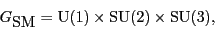while the fundamental fermions and their antiparticles are basis vectors of a highly reducible representation of this group, which we call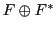. Here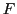describes the fermions, while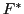describes their antiparticles.

Amazingly, using the ideas of gauge theory and quantum field theory, plus the Higgs mechanism' for symmetry breaking, we can recover the dynamical laws obeyed by these particles from the representation of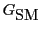on. This information is enough to decode the physics of these particles and make predictions about what is seen in the gigantic accelerators that experimental physicists use to probe the natural world at high energies. Unfortunately, to explain all this would go far beyond the modest goals of this paper. For a guide to further study, see Section 1.1.

Having acquainted the reader with the Standard Model of particle physics in Section 2, we then go on to talk about grand unified theories in Section 3. These theories go beyond the Standard Model by extending' the gauge group. That is, we pick a way to includein some larger group, and choose a representationofwhich reduces to the Standard Model representationwhen we restrict it to. We describe how this works for thetheory (Section 3.1), thetheory (Section 3.2), and the Pati-Salam model (Section 3.3). Of course, since we do not discuss the dynamics, a lot will go unsaid about these GUTs.

As we proceed, we explain how thetheory and the Pati-Salam model are based on two distinct visions about how to extend the Standard Model. However, we will see that thetheory is an extension of both thetheory (Section 3.2) and the Pati-Salam model (Section 3.4). Moreover, these two routes to thetheory are compatible in a precise sense: we get a commuting square of groups, and a commuting square of representations, which fit together to form a commuting cube (Section 3.5).

In Section 4, we conclude by discussing what this means for physics: namely, how the Standard Model reconciles the two visions of physics lying behind thetheory and the Pati-Salam model. In a sense, it is the intersection of thetheory and the Pati-Salam model within their common unification,.

Throughout the course of the paper, we occasionally summarize our progress in theorems, most phrased in terms of commutative diagrams:

• Section 3.1, Theorem 1: thetheory extends the Standard Model.
• Section 3.2, Theorem 2: thetheory extends thetheory.
• Section 3.3, Theorem 3: the Pati-Salam model extends the Standard Model.
• Section 3.4, Theorem 4: the Pati-Salam model is isomorphic to a theory involving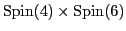.
• Section 3.4, Theorem 5: thetheory extends the Standard Model.
• Section 3.4, Theorem 6: thetheory extends thetheory.
• Section 3.5, Theorem 7: the Standard Model, thetheory, thetheory and thetheory fit together in a commutative cube.
• Section 4, Theorem 8: The true gauge group of the Standard Model is the intersection ofand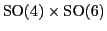in.
• Section 4, Theorem 9: The true gauge group of the Standard Model is the intersection ofand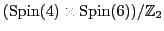in.

Subsections
2010-01-11